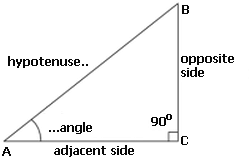### Right Triangle

The Triangle is a plane closed figure composed by 3 straight lines that do not intersect, but are joined at their extremities.
All the right triangle has one of the angles measuring 90 degrees or PI/2 radians. In a right triangle, taken as base, one of its sides, except for the side "c", known as the hypotenuse, the height is equal to another side, perpendicular to the base.
Read full text in Right Triangle.
To calculate Right triangle enter the requested information, using the point as a decimal separator. Ex 1,250.37 enter: 1250.37 , the results will be shown after a click on CalculateRight Triangle Decimal places: Opposite Side  Adjacent Side Adjacent Side Hypotenuse Perimeter: Area: Angle A: Angle B: Angle C: Altitude base AB: Altitude base AC: Altitude base BC: Incircle radius: Excircle radius: Nota:

Note: The accuracy of the calculator and its applicability to particular cases is not guaranteed.